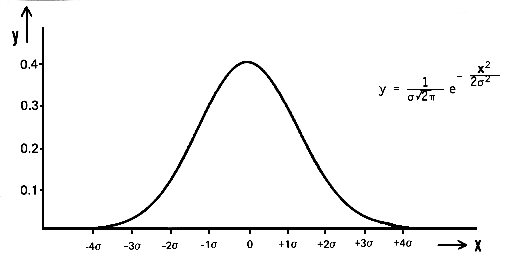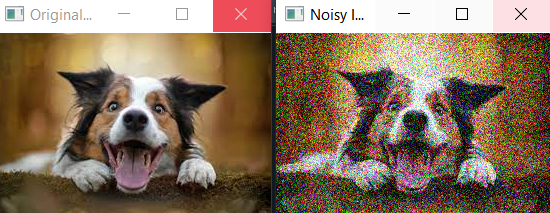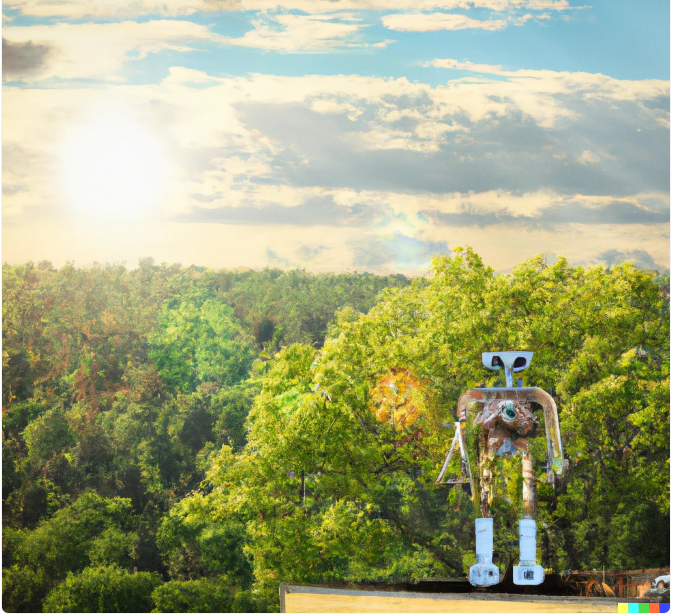# What is Gaussian Noise in Deep Learning? How and Why it is used?Close Up Photo of Toy Bot - Free Stock Photo (pexels.com)

### What is Gaussian Noise in Deep Learning? How and Why it is used?

In a mathematical way, Gaussian noise is a type of noise that is generated by adding random values that are normally distributed with a mean of zero and a standard deviation (σ) to the input data. The normal distribution, also known as the Gaussian distribution, is a continuous probability distribution that is defined by its probability density function (PDF):

``````pdf(x) = (1 / (σ _ sqrt(2 _ π))) * e^(- (x --- μ)² / (2 * σ²))
``````https://www.sfu.ca/sonic-studio-webdav/handbook/Gaussian_Noise.html

#### where x is the random variable, μ is the mean, and σ is the standard deviation.

The Gaussian noise can be added to the input data or weights by generating random values with a normal distribution and adding them to the input data or weights.

For example, if we want to add Gaussian noise to an image, we can represent the image as a 2D matrix of pixel values, and then generate random values with a normal distribution using numpy library `np.random.randn(rows,cols)` , and add them to the pixel values of the image. This will result in a new image with added Gaussian noise.

Similarly, if we want to add Gaussian noise to the weights of a deep learning model, we can generate random values with a normal distribution, and add them to the weights during the training process.

Gaussian noise, also known as white noise, is a type of random noise that is distributed according to a normal distribution. In deep learning, Gaussian noise is often added to the input data during training to improve the robustness and generalization ability of the model. This is known as data augmentation. By adding noise to the input data, the model is forced to learn features that are robust to small variations in the input, which can help it perform better on new, unseen data. A

### Lets start with a simple and Real Example as below first, so you can have feel what we are talking about:

In this example, the standard deviation of the noise (`noise_std`) is set to a larger value of 50, which will result in more noise being added to the image. You can see that the noise is more pronounced, and the features of the original image are less distinct.

It's worth noting that when adding more noise, it's important to ensure that the noise does not exceed the valid range of pixel values (i.e. between 0 and 255). In this example, the `np.clip()` function is used to ensure that the pixel values of the noisy image fall within the valid range.

It's also important to keep in mind that while more noise may make it easier to see the difference between the original and noisy images, it may also make it more difficult for the model to learn useful features from the data, and may lead to overfitting or underfitting. It's usually a good idea to start with a small amount of noise and gradually increase it while monitoring the performance of the model.

``````import cv2
import numpy as np

# Add Gaussian noise to the image

noise*std = 50
noise = np.random.randn(_image.shape) * noise_std
noisy_image = np.clip(image + noise, 0, 255).astype(np.uint8)

# Display the original and noisy images

cv2.imshow('Original Image', image)
cv2.imshow('Noisy Image', noisy_image)
cv2.waitKey(0)
cv2.destroyAllWindows()
``````Program Output

### Some examples of how Gaussian noise is used in deep learning.

1. Data augmentation: One common use of Gaussian noise in deep learning is to add it to the input data during training. For example, if you're training a model to recognize images of handwritten digits, you might add Gaussian noise to each image before passing it through the model. This would force the model to learn features that are robust to small variations in the input, such as a smudge on the image or slight misalignment. As a result, the model would be more likely to correctly recognize the digit even if the image is slightly different from the training data.
2. Weight Decay: Gaussian noise can be added to the weights of a neural network during training as a form of regularization, though this is less common compared to other methods such as L1/L2 regularization or dropout. This is known as weight noise or weight decay.Adding Gaussian noise to the weights during training is similar to injecting noise to the inputs, both aim to increase the robustness of the model. It forces the network to not rely too heavily on any single weight and helps the model to generalize better by preventing overfitting. The idea behind this is that small random perturbations of the weights can create a form of stochasticity that helps to smooth the loss landscape, and can act as a form of implicit regularization.
3. Regularization: Adding Gaussian noise to the parameters of a model can also be seen as a regularization technique. It forces the model to have smaller weights values, which in turn make the model more general and less prone to overfitting.
4. Adversarial training: In adversarial training, the model is trained on examples that are augmented with small, targeted perturbations, such as Gaussian noise. This makes the model more robust to adversarial examples, which are inputs that have been specifically crafted to fool the model.
5. Semi-supervised learning: Gaussian noise can be added to the input data during training to improve the performance of semi-supervised models. This can help the model to better leverage the limited labeled data and learn more general features.
6. Transfer learning: Gaussian noise can be added to the input data during fine-tuning to improve the performance of transfer learning models. This can help the model to better adapt to the new task and generalize better to unseen data.
7. Generative Adversarial Networks (GANs): Gaussian noise can be added to the generator input to improve the diversity of the generated samples.
8. Bayesian deep learning: Gaussian noise can be added to the weights of a model during training to make it more robust to overfitting and improve the generalization ability of the model.
9. Reinforcement learning: Gaussian noise can be added to the input or action space of an agent during training to make it more robust to variations in the environment and improve the generalization ability of the agent.

In all of the above examples, Gaussian noise is added to the input or weights in a controlled manner, with a specific mean and standard deviation. The goal is to improve the model's performance and robustness without making it too difficult for the model to learn from the data.

### Lets Discuss some of how to add Gaussian noise to the input data during training using Python and the popular deep learning library Keras.

I will not go into each case as it will take many blogs to jsut go with one example in detail, Lets have a complete overview today over gaussian Noise and its application

Here is an example of how to add Gaussian noise to the input data before passing it through the model during training:

``````from keras.preprocessing.image import ImageDataGenerator

# Define the data generator

datagen = ImageDataGenerator(
featurewise_center=False, # set input mean to 0 over the dataset
samplewise_center=False, # set each sample mean to 0
featurewise_std_normalization=False, # divide inputs by std of the dataset
samplewise_std_normalization=False, # divide each input by its std
zca_whitening=False, # apply ZCA whitening
rotation_range=0, # randomly rotate images in the range (degrees, 0 to 180)
width_shift_range=0.1, # randomly shift images horizontally (fraction of total width)
height_shift_range=0.1, # randomly shift images vertically (fraction of total height)
horizontal_flip=False, # randomly flip images
vertical_flip=False, # randomly flip images
noise_std=0.5 # add gaussian noise to the data with std of 0.5
)

# Use the generator to transform the data during training

model.fit_generator(datagen.flow(x_train, y_train, batch_size=32),
steps_per_epoch=len(x_train) / 32, epochs=epochs)
``````

Here, the `ImageDataGenerator` class from Keras is used to define a data generator that applies the specified data augmentation techniques to the input data. In this case, we set `noise_std` to 0.5, which means that Gaussian noise with a standard deviation of 0.5 will be added to the input data. The generator is then used during the call to `model.fit_generator` to apply the data augmentation to the input data during training.

### Lets see more examples of how to add Gaussian noise to the input data and weights during training using Python and the popular deep learning library Keras.

NOTE: These examples are not meant to be complete, production-ready code, but rather simplified snippets to illustrate the concepts.

For adding noise to the input data, we can use the `numpy` library to generate random noise and add it to the input data. Here's an example of how to do this:

``````import numpy as np

# Generate some random input data

x_train = np.random.rand(1000, 64)
y_train = np.random.rand(1000, 10)

# Add Gaussian noise to the input data

noise_std = 0.5
x_train_noisy = x_train + noise_std * np.random.randn(*x_train.shape)

# Train the model

model.fit(x_train_noisy, y_train, epochs=10)
``````

In this example, the input data `x_train` is a 2D array of shape (1000, 64) and the noise is generated using `np.random.randn(*x_train.shape)` which will return an array of the same shape with random values that are normally distributed with mean 0 and standard deviation 1. Then we add the generated noise to the input data by multiplying it with the standard deviation of the noise (0.5) and adding it to the input data.

### Gaussian noise is a widely used technique in deep learning and has a number of applications. Here are a few examples:

1. Image classification: Gaussian noise can be added to images during training to improve the robustness of image classification models. This is particularly useful when the training data is limited or has a lot of variability, as the model is forced to learn features that are robust to small variations in the input.

Here's an example of how to add Gaussian noise to images during training to improve the robustness of an image classification model:

``````from keras.preprocessing.image import ImageDataGenerator

# Define the data generator

datagen = ImageDataGenerator(
featurewise_center=False, # set input mean to 0 over the dataset
samplewise_center=False, # set each sample mean to 0
featurewise_std_normalization=False, # divide inputs by std of the dataset
samplewise_std_normalization=False, # divide each input by its std
zca_whitening=False, # apply ZCA whitening
rotation_range=0, # randomly rotate images in the range (degrees, 0 to 180)
width_shift_range=0, # randomly shift images horizontally (fraction of total width)
height_shift_range=0, # randomly shift images vertically (fraction of total height)
horizontal_flip=False, # randomly flip images
vertical_flip=False, # randomly flip images
noise_std=0.5 # add gaussian noise to the data with std of 0.5
)

# Use the generator to transform the data during training

model.fit_generator(datagen.flow(x_train, y_train, batch_size=32),
steps_per_epoch=len(x_train) / 32, epochs=epochs)
``````

2. Object detection: Similarly, Gaussian noise can be added to the input data during training of object detection models to make them more robust to small changes in the image such as lighting conditions, occlusions and camera angles.

Example of how to add Gaussian noise to the input images during training to improve the robustness of an object detection model

``````def add*noise(image, std):
"""Add Gaussian noise to an image."""
noise = np.random.randn(image.shape) * std
return np.clip(image + noise, 0, 1)

# Add noise to the training images

x_train_noisy = np.array([add_noise(img, 0.1) for img in x_train])

# Train the model

model.fit(x_train_noisy, y_train, epochs=10)
``````

3. Speech recognition: Gaussian noise can be added to audio data during training to improve the robustness of speech recognition models. This can help the model to better handle background noise and other disturbances in the audio signal.

Example of how to add Gaussian noise to audio data during training to improve the robustness of a speech recognition model:

``````def add*noise(audio, std):
"""Add Gaussian noise to an audio signal."""
noise = np.random.randn(audio.shape) * std
return audio + noise

# Add noise to the training audio

x_train_noisy = np.array([add_noise(audio, 0.1) for audio in x_train])

# Train the model

model.fit(x_train_noisy, y_train, epochs=10)
``````

4. Generative models: In generative models such as GANs, Generative Pre-training Transformer (GPT), and VAEs, Gaussian noise can be added to the input data during training to improve the ability of the model to generate new, unseen data.

Example of how to add Gaussian noise to the input data during training to improve the ability of a GAN

``````# Generate random noise

noise = np.random.randn(batch_size, 100)

# Generate fake images

fake_images = generator.predict(noise)

# Add Gaussian noise to the fake images

fake_images_noisy = fake_images + 0.1 * np.random.randn(*fake_images.shape)

# Train the discriminator

discriminator.train_on_batch(fake_images_noisy, np.zeros((batch_size, 1)))
``````

In this example, the generator is trained to generate new images based on random noise as input, and Gaussian noise is added to the generated images before they are passed to the discriminator. This improves the ability of the generator to generate new, unseen data.

5. Adversarial training: Gaussian noise can be added to the input data during adversarial training to make the model more robust to adversarial examples.

Below is the Example of how to add Gaussian noise to the input data during adversarial training to make the model more robust to adversarial examples.In this example, the Fast Gradient Sign Method (FGSM) is used to generate adversarial examples, and Gaussian noise is added to the adversarial examples before they are passed to the model during training. This improves the model's robustness to adversarial examples.

``````# Generate adversarial examples

x_adv = fgsm(model, x_train, y_train, eps=0.01)

noise_std = 0.05

# Train the model

``````

6. Denoising: Gaussian noise can be added to an image or signal and the goal of the model is to learn to remove the noise and restore the original signal.In this example, the input images `x_train` are first corrupted with Gaussian noise with a standard deviation of 0.1 and then the corrupted images are passed through the denoising autoencoder to reconstruct the original images. The autoencoder learns to remove the noise and restore the original signal.

``````# Add Gaussian noise to the images

noise_std = 0.1
x_train_noisy = x_train + noise_std * np.random.randn(*x_train.shape)

# Define the denoising autoencoder

input_img = Input(shape=(28, 28, 1))
x = Conv2D(32, (3, 3), activation='relu', padding='same')(input_img)
x = Conv2D(32, (3, 3), activation='relu', padding='same')(x)

# at this point the representation is (7, 7, 32)

x = Conv2D(32, (3, 3), activation='relu', padding='same')(encoded)
x = UpSampling2D((2, 2))(x)
x = Conv2D(32, (3, 3), activation='relu', padding='same')(x)
x = UpSampling2D((2, 2))(x)
decoded = Conv2D(1, (3, 3), activation='sigmoid', padding='same')(x)

autoencoder = Model(input_img, decoded)
``````

7. Anomaly detection: Gaussian noise can be added to normal data and the goal of the model is to learn to detect the added noise as an anomaly.

``````# Add Gaussian noise to the normal data

noise_std = 0.1
x_train_noisy = x_train + noise_std * np.random.randn(*x_train.shape)

# Concatenate the normal and the noisy data

x_train_concat = np.concatenate((x_train, x_train_noisy))
y_train_concat = np.concatenate((np.zeros(x_train.shape), np.ones(x_train_noisy.shape)))

# Train the anomaly detection model

model.fit(x_train_concat, y_train_concat, epochs=10)
``````

8. Robust optimization: Gaussian noise can be added to the parameters of a model during optimization to make it more robust to small perturbations in the parameters.

``````# Define the loss function

def loss_fn(params):
model.set_weights(params)
return model.evaluate(x_test, y_test, batch_size=32)

# Define the optimizer

# Define the step function

def step_fn(params):
loss = loss_fn(params)
return params + noise_std * np.random.randn(*params.shape)

# Optimize the model

params = model.get_weights()
for i in range(100):
``````

### Summary:

Gaussian noise is a technique used in deep learning to add randomness to the input data or weights. It is a type of noise that is generated by adding random values that are normally distributed with a mean of zero and a standard deviation (σ) to the input data. The goal of adding noise to the data is to make the model more robust to small variations in the input and better able to handle unseen data. Gaussian noise can be used in a wide range of applications, such as image classification, object detection, speech recognition, generative models, and robust optimization. For example, in image classification, Gaussian noise can be added to images during the training to improve the robustness of the models, this can be visualized by comparing the original images with the images with added Gaussian noise. The model learns to recognize the features of the image despite the added noise, and becomes more robust to small variations in the input.Ddall E